## Typecho新年灯笼插件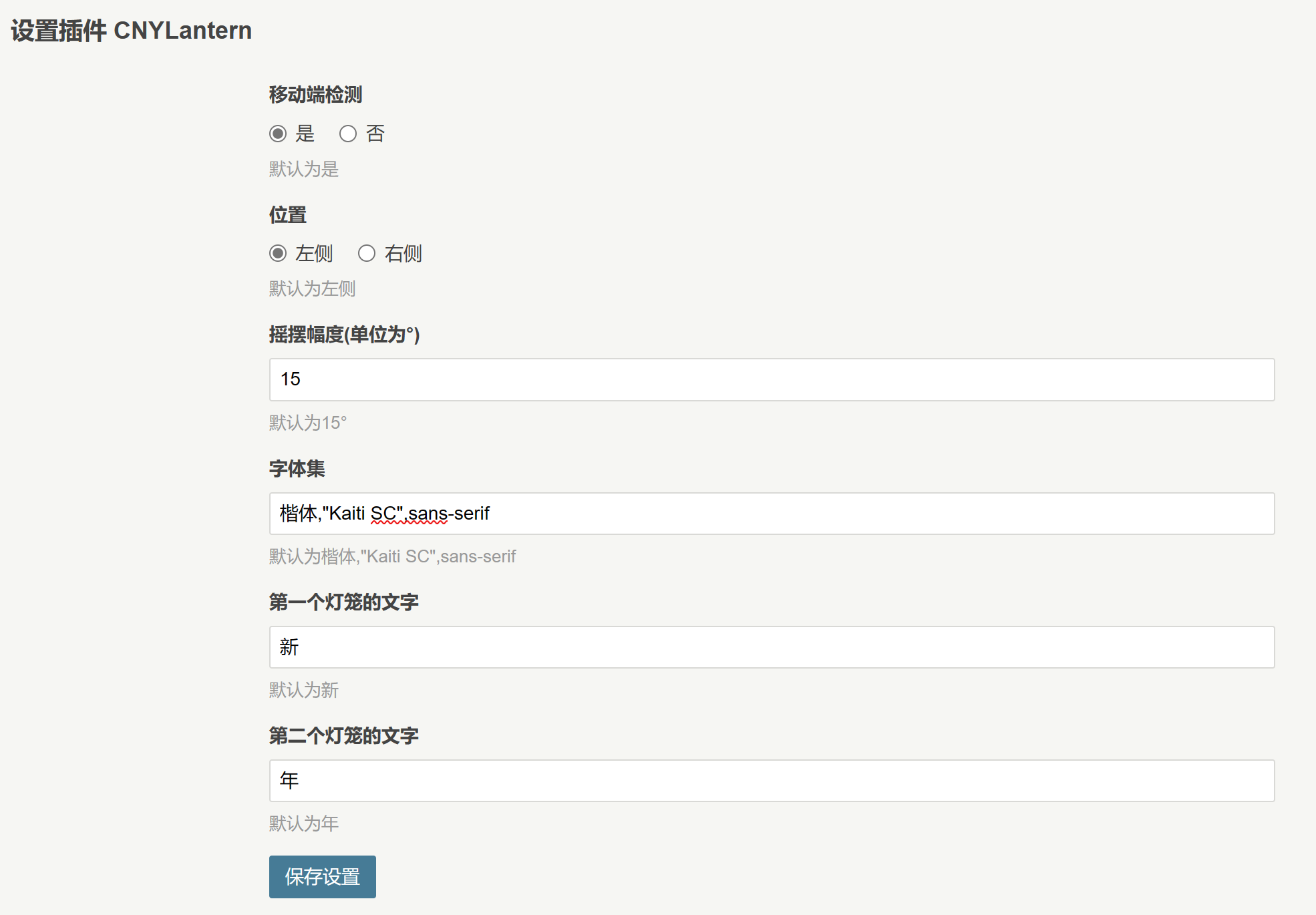## 更新日志与下载

1.2R->1.3R
<FEB.14-2023>
CNYLtn_1.3R.zip
1.添加调试模式，开启后可在前台控制台中输出调试信息
2.整体结构优化

1.1R->1.2R
<JAN.11-2023>
CNYLtn_1.2R.zip
1.修复在英文版Windows系统和MacOS下的默认字体回归问题
2.修改组件动画显示
3.修改字体大小为3em并在灯笼中居中

1.0R->1.1R
<JAN.04-2023>
CNYLtn_1.1R.zip
1.修复移动端检测无效的问题

1.0R
<JAN.03-2023>

## 代码段

``````#include <iostream>

int main() {
auto string = "Hello, World! ";
auto integer = 114514;
std::cout << string << integer << std::endl;
system("pause");
return 0;
}``````

## 入口点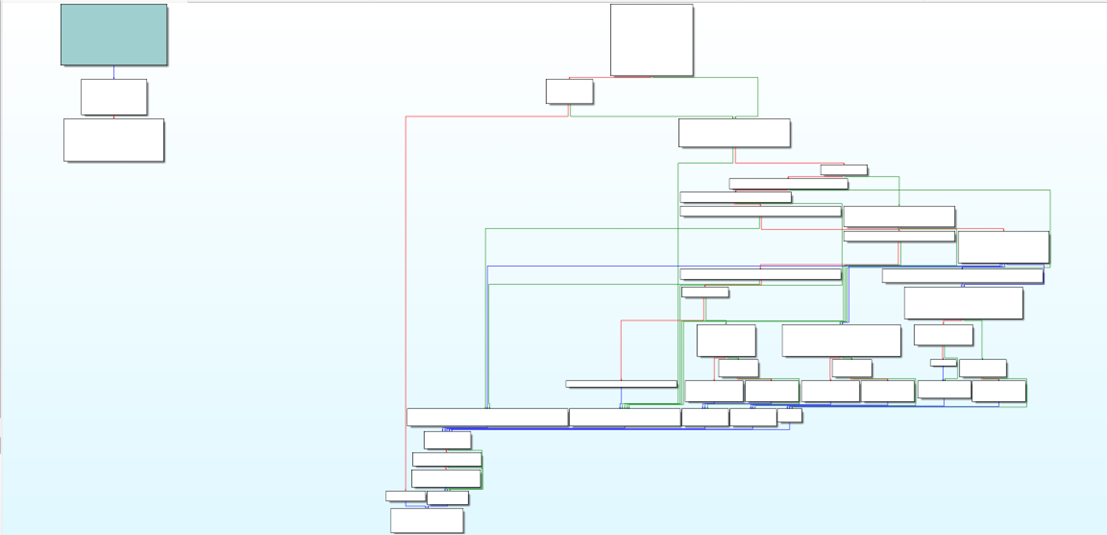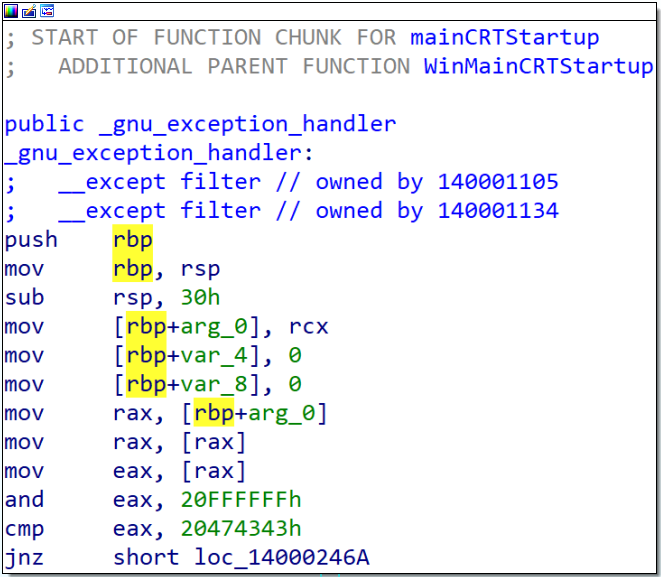## main?WinMainCRTStartup和mainCRTStartup?

WinMainCRTStartup和mainCRTStartup是CRT（C Runtime）库提供的启动代码。它们都是程序入口点，但是它们的参数和返回值类型不同，适用于不同的应用程序类型以及适配了不同平台的实现。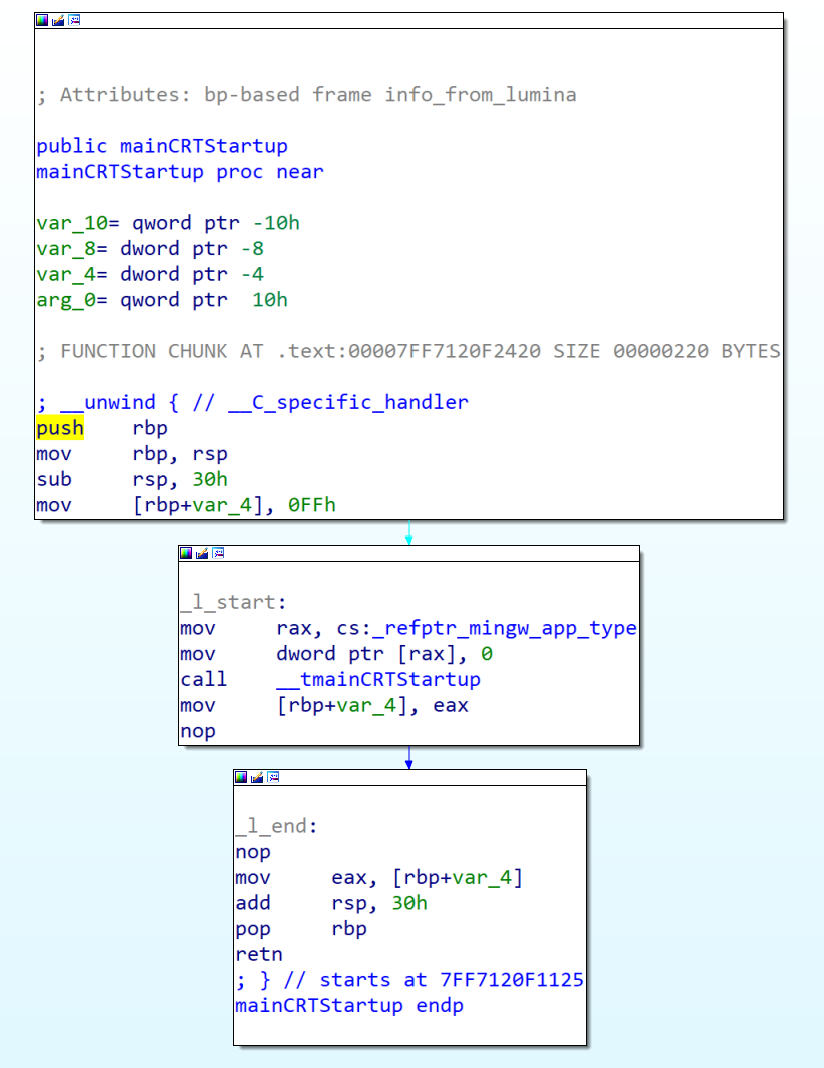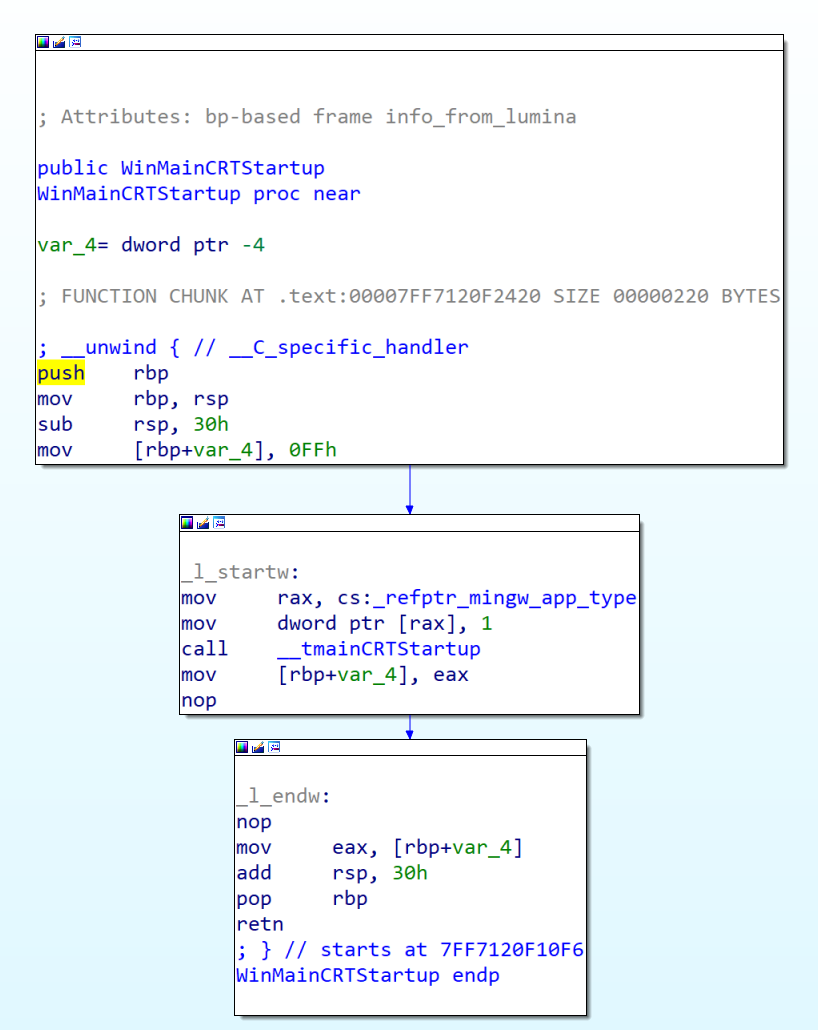## 反编译的伪代码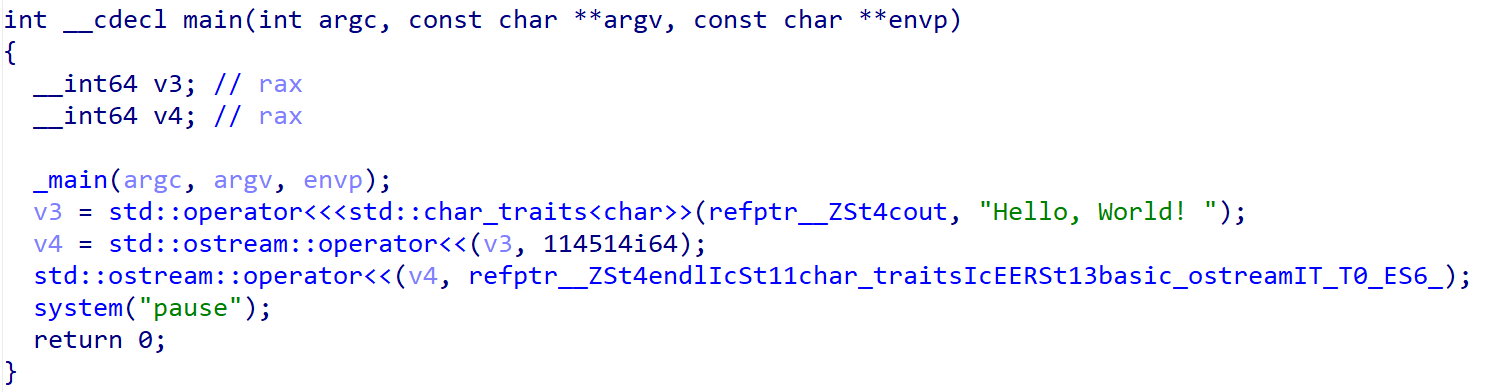## 总结

1.初始化C/C++运行时环境，包括初始化全局变量、静态变量等。
2.调用MainCRTStartup/WinMainCRTStartup函数，该函数初始化Win32 API环境，并调用Main函数。
3.设置标准输入、标准输出和标准错误流，以便程序可以向控制台输出信息。
4.初始化异常处理机制，包括设置SEH（结构化异常处理）机制*Windows特有。

## S社NB30機種向ATCmd

C#写的首个练手项目

``````/* NB30 AtCmdXSoftUpdate v0.1
(C)Yuu 2020
*/
using System;
using System.Collections.Generic;
using System.Text;
using System.IO.Ports;

namespace AtCmdXSoftUpdate {
class Program {
static void Main(string[] args) {
Console.WriteLine("Nb30 AtCmdXSoftUpdate v0.1");
SerialPort serialPort = new SerialPort();
serialPort.PortName = "COM30";
serialPort.BaudRate = 115200;
serialPort.DataBits = 8;
serialPort.Parity = Parity.None;
serialPort.StopBits = StopBits.One;
serialPort.Handshake = Handshake.None;
serialPort.WriteTimeout = 500;
serialPort.Open();

serialPort.WriteLine("AT+XSOFTUPDATE");
if (true == response.Contains("OK")) {
} else {
Console.WriteLine("ERROR");
}
serialPort.Close();
}
}
}``````

## S社NB30機種向SHDIAG

``````import sys
import usb.core
import usb.util
import random

NB30_USB_VID = 0x04dd
NB30_USB_PID = 0x933a

def shdiag_xchar(c):
xft = [0, 2, 4, 6, 1, 3, 5, 7]
r = 0
for i in range(8):
r |= (((c >> i) & 1) << xft[i])
return r

def shdiag_encode_data(in_data):
ip = 0
op = 1
out_data =  * (len(in_data) * 2)
v = (random.randint(0, 0x7f) + 0xf)
x = shdiag_xchar(v)
out_data = v

for char in in_data:
if char in '\r\n':
out_data[op] = ord(char)
op += 1
if char == '\n':
x = shdiag_xchar(v)
out_data[op] = v
op += 1
else:
out_data[op] = (ord(char) ^ (x & 0x7f)) + 0xf
x = (x >> 1) | ((x & 1) << 7)
op += 1

return out_data[:op]

def shdiag_decode_data(in_data):
ip = 1
op = 0
out_data =  * (len(in_data) - 1)
x = shdiag_xchar(in_data)

for char in in_data[1:]:
if char in '\r\n':
out_data[op] = char
op += 1
if char == '\n':
x = shdiag_xchar(in_data[ip + 1])
else:
out_data[op] = (char - 0xf) ^ (x & 0x7f)
x = (x >> 1) | ((x & 1) << 7)
op += 1

return out_data[:op]

def nb30port_open():
dev = usb.core.find(idVendor=NB30_USB_VID, idProduct=NB30_USB_PID)
if dev is None:
dev.set_configuration()
cfg = dev.get_active_configuration()
intf = cfg[(1, 1)]
ep_in = usb.util.find_descriptor(intf, custom_match=lambda e: usb.util.endpoint_direction(
ep_out = usb.util.find_descriptor(intf, custom_match=lambda e: usb.util.endpoint_direction(
return dev, ep_in, ep_out

def nb30port_close(dev):
usb.util.dispose_resources(dev)

def nb30port_write(ep_out, data):
return ep_out.write(data, 1000)

def main():
if len(sys.argv) != 2:
print("YuuSHDIAG v0.2")
print("Usage: python shdiag.py <SHDIAG_COMMAND>")
return 1

dev, ep_in, ep_out = nb30port_open()

if res:
res_decode = shdiag_decode_data(res)
print(''.join(map(chr, res_decode)))

req = '{}\r\n'.format(sys.argv)
req_encode = shdiag_encode_data(req)
if req_encode and len(req_encode) == nb30port_write(ep_out, req_encode):
if res:
res_decode = shdiag_decode_data(res)
print(''.join(map(chr, res_decode)))

nb30port_close(dev)

if __name__ == '__main__':
try:
main()
except Exception as e:
print(str(e))
``````